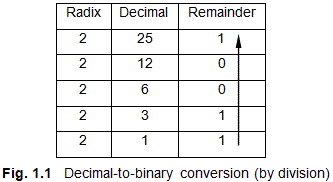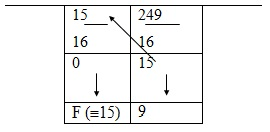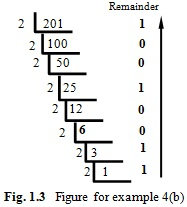## Wednesday, 30 November 2016## Conversion from Decimal to Binary Fractions

In the decimal system, fractions are represented as 0. abc. . . , where a, b, c, etc. denote any digit in between 0 and 9.  The decimal point separates the integral part of the number from its fractional part. Consider the number (16.346)10. The expansion of this number is as follows:

Integral part:    (16)10     =  1 ´ 101 + 6 ´ 100
Fraction:    (0.346)10 =  3 ´ 10–1 + 4 ´ 10–2 + 6 ´ 10–3
=  3/10 + 4/100 + 6/1000

As shown above, in the case of fractions also, we are using the coefficients of the negative power of 10 to express fractional numbers. This means that the numbers after the point symbol are expanded by using negative powers of 10. The same concept can be used in all the number systems for representing fractions. For example, consider the binary number (11.101)2. This may be expanded as:

Integral part: (11)2      = 1 ´ 21 + 1 ´ 20 = (3)10
Fraction: (0.101)2  = 1 ´ 2–1 + 0 ´ 2–2 + 1 ´ 2–3 = 0.5 + 0.125 = (0.625)10

The decimal equivalent of the binary number (11.101)2 is thus obtained as:

(11.101)2 = 3 + 0.625 = (3.625)10

It may be noted that the point symbol in the binary system may be called as the binary point. In general, this may be designated as radix point.

Conversion from Decimal Fraction to Binary Fraction

Just as we divided a decimal whole number by the factor 2 to get its binary-equivalent whole number, to get the corresponding binary fraction we multiply decimal fraction also by 2. If the product of each number is greater than 1, we put a 1 below that number; otherwise we put a 0 as shown in Table 1.2.

Example 4: Convert decimal fraction 0.875 into its equivalent binary fraction.

Solution: Following the directions given above, we first draw the decimal fraction-to-binary fraction conversion table as illustrated in Table 1.2. One point to be remembered here is that multiplication of fractional part by 2 must be conducted from left-to-right (for the integral part, division by 2 has to be done from right-to-left as described in Example 3).

It can be seen that the multiplication operation stops at the third position on the right because the remainder at this point is 0. If the remainder is not 0, then multiplication by 2 must continue up to that point where the remainder becomes 0. However, if the remainder does not become 0, then we get a continued fraction. This is illustrated in the next example.

(0.875)10 ≡ (0.111)2

Example 5: Convert the decimal fraction 0.862 into its equivalent binary fraction.

Solution: Proceeding as explained in Example 4, we prepare Table 1.3, the decimal fraction-to-binary fraction table as shown. We find from Table 1.3 that the fraction does not converge; it continues as (110110…)2. We conclude that a binary fraction will converge only if and only if the last number in the expanded decimal fraction is 0.5, so that 0.5 ´ 2 = 1; otherwise the fraction will continue From Table 1.3, we find that

From Table 1.2, we find that
(0.862)10 ≡ (0.110110…)2

Conversion of Mixed Decimal Number-to-Mixed Binary Number

Example 6: Convert the decimal number (26.012) into equivalent binary number.

Solution: We first convert 26 into binary whole number, and then (0.012)10 into binary fraction.  This is done as shown in Table 1.4.

From table 1.4, we obtain the binary equivalent of decimal number 26 as 11010. Next, we consider the fractional part. This is done as shown in Table 1.5. From Table 1.5, we find that the binary equivalent of decimal fraction 0.6875 is 0.1011.

Combining the integer and fractional parts, we get

(26.6875)10  = (11010.1011)2## Decimal to Binary Example Problems

Two methods are used for converting decimal numbers to their equivalent binary numbers.

Conversion Using Division

Example 1: Convert (25)10 into equivalent binary number.

Solution:  The procedure using division is illustrated in Fig.1.1.  In this, we divide the given number repeatedly by 2. In each division, if the number is exactly divisible by 2, we put a 0 as remainder against the number on its right-hand side. If the number is not exactly divisible by 2, we put a 1 as the remainder. We proceed like this up to the last digit, as shown in Fig. 1.1. We then read upwards, in the direction of the arrow shown to get the required binary number.

From the Fig. 1.1, we find the binary equivalent of decimal number as

(25)10 = (10011)2­

Check:  (11001)2 = 1 ´ 24 + 1 ´ 23 + 0 ´ 22 + 0´21+ 1 = 16 + 8 + 1  = (25)10

Example 2: Convert decimal numbers (a) 221 and (b) 10120 into equivalent binary numbers.

Solution: Instead of going into long division process, a very easy and simple method of decimal-to- binary conversion is given below.
(a) In this method, we first find the powers of 2 which add up to yield the given number. We know that 27 = 128 and 28 = 256. Since 221 is less than 256, we conclude that 221 = 128 + some numbers which are lower powers of 2. To find these, we subtract 128 from 221, which yields

221 ‒ 128 = 93

The nearest power of 2 is 64. Subtracting this from 93, we get

93 ‒ 64 = 29

Proceeding in this way, successive subtraction shows that

29 = 16+8+4+1

Thus the given number is decimated as:

221 =128+64+0×32+16+8+4+0×2+1 = 11011101

where we have substituted 0s in the places of 32 and 2, respectively, as these two numbers are absent in the summation.
(b) Decimating 10120, we find that”

10120 = 8192+1024+512+256+128+8
= 10001110001000
Conversion by Tabulation

This is an extension of the fist method explained illustrated in Example 1.

Example 3: Convert decimal number (83)10 into its binary equivalent by the tabulation method.

Solution: In this method, we first prepare Table 1.1, which is the decimal-to-binary conversion table. Table 1.1 has 7 columns (this number depends on the number of divisions we have to perform and 4 rows (fixed).

In Table 1.1, the first step is to enter the decimal number to be converted into binary (in this case 83) into Column 1 of Row 1 as shown. The second step is to enter the radix of the number system to which conversion is required into all the columns of Row 2. Here, conversion is required into the binary system and hence its radix 2 is entered into all the columns of Row 2, as stated.

The third step is to divide 83 by radix number 2. We notice that 83 is not fully divisible by 2. 83 divided by 2 yields 42 as quotient and 1 as remainder. These are the respective entries in column 1 of Rows 3 and 4. Summarizing the operations described above, we find that the entry under Column 1, Row 1 is 83, Row 2 is 2, Row 3 is 42, and Row 4 is 1.

The next step is to enter number 42, the quotient of the first division, into Column 2, Row 1 as shown in Table 1.1. Proceeding as above, 42 is divided by radix 2. This operation yields a quotient of 21, and a remainder of 0; we enter 21 in Row 3 and 0 in Row 4 under Column 2 of table 1.1, as shown. In the table, we have used slashed arrows to indicate the transfer of quotient numbers and vertical arrows to denote the direction of division operation. For example, the first and rightmost slashed arrow indicates the transfer of the first quotient number 42 to Column-2, Row-1 cell, as shown in Table 1.1. The first vertical arrow 1 indicates that division of 83 by 2 gives 42 as the quotient and 1 as the remainder. These vertical transfers are also shown in Table 1.1.

We find that 42 becomes the next (second) entry to be divided by radix 2. The division yields the quotient as 21 and remainder as 0, since 42 is fully divisible by 2. As the next step, we divide 21 by 2 in Column 3. The results of this and subsequent operations described above are indicated in Table 1.1. We stop the division and associated operations in Column 7 (for this problem) when the remainder becomes 1 and no further division is possible.

Reading the entries in Row 4 (remainder row), rightwards, we find

(83)10­ = (1100101)2

It can be seen that this method is very convenient for conversion purposes, if the decimal number is less than 100 or 200. Above this range, the table size becomes too unwieldy. However, this method becomes quite convenient for converting octal to hexadecimal numbers

## Tuesday, 29 November 2016Hexadecimal number system uses 16 as its base or radix. The basic elements of this system consist of digits from 0 to 9 and alphabets A, B, C, D, E, and F. Letter A to F are assigned with values of 10 to 15. Thus A ≡ 10, B ≡ 11, C ≡ 12, D ≡ 13, E ≡ 14, and F ≡ 15. In terms of binary equivalents, A ≡ 1010, B ≡ 1011, C ≡ 1100, D ≡1101, E ≡ 1110, and F ≡ 1111. Hexadecimal system is used in microprocessors and microcontrollers to write their operating codes (op-codes). Since there are sixteen basic numbers to play with, instructions using hex are more compact than those the using decimal or binary number systems.

Decimal Number to Hexadecimal Number Conversion:
Example: Convert (249)10 to hex.
Solution: (See Fig.1.13)Fig.1.13   Conversion of (249)10 to HEX
From Fig.1.13, we have
(249)10 º (F9)16
The 9’s-complement pairs in this family are (0,9), (1,8), (2,7), (3,6) and (4,5), respectively, since the sum of the numbers in each bracket amounts to 9.  We also get the 10’s complements by adding +1 to the 9’s complements. For example, 4 is the 10’s complement of 6.## The Binary Number System

THE BINARY NUMBER SYSTEM

In the binary number system, 0 and 1 are the basic elements. Since there are only two digits in this scheme, we say that its radix is 2. The entire number system is built upon based on these two numbers. We call 0 and 1 as binary digits or bits when they are used in the binary number system.  0 and 1, as stated above, can be used to represent any number in binary system.  For example, (1101)2 represents a binary number, where the digits 1, 1, 0, and 1, represent coefficients of appropriate powers of 2. Thus For example, 1101 is a short form of writing, whose expansion is:

(1101)2 = (1*23) + (1*22) + (0*21) + (1*20)

Decimal equivalent of 1101)2 can be obtained by converting the powers of 2 to their respective decimal values. Thus

(1101)2 = (1*8) + (1*4) + (0*2) + 1 = 8 + 4+ 1 = (13)10

The procedure given above can be used to convert any binary number into its corresponding decimal number.## The Decimal Number System

Modern digital technology is dependent entirely upon the binary number system, which has only two elements, viz., 0 and 1, in it. However, we are more familiar with decimal number system, which has ten basic elements (0 to 9) in it. In this chapter, we discuss the principles of various number systems that we commonly come across in various contexts. The discussion begins with the decimal number system. This is followed by the binary, octal, and hexadecimal number systems.

THE DECIMAL NUMBER SYSTEM

The decimal number system is the most commonly and widely used number system by the common man. As stated above, we have ten elemental numbers in this number system. They are, respectively, 0, 1, 2, 3, 4, 5, 6, 7, 8, and 9. Since there are ten digits in this group, we say that the number system has a base or radix of 10. In the decimal number system, numbers greater than 9 are represented by repeatedly using these digits in a definite order. As an example, consider let us consider the decimal number 123. This may be expressed in the form (123)10, where the subscript 10 represents the base or radix of number 123. It can be seen that 123 represents a short form of expressing numbers. This may  be expanded as:

(123)10  = (1 ´ 102) + (2 ´ 101) + (3 ´ 100)

where digits 1, 2, and 3 are the coefficients of the appropriate powers of ten (i.e., 102, 101, and 100, respectively). Larger numbers can be expressed in similar fashion by writing them as coefficients of the powers of ten. In fact, in any number system, numbers are expressed as coefficients of appropriate powers of the radix of the system.

Decimal Number to Binary Number Conversion:

We proceed in a similar fashion, as given above. This is shown in Fig.1.3.
From Fig.1.3, we get

(201)10 = (11001001)2

Decimal Number to Octal Number Conversion:

Example: Convert the decimal number 1234 into its equivalent octal number.

Solution:  Figure 1.9 shows a conversion table prepared on the basis of the steps shown in Fig. 1.8.Fig. 1.9 Tabular form for converting (1234)10 into octal

From Fig. 1.9, we find that

(1234)10 º (2322)8
Check:   2 ´ 83 + 3 ´ 82 + 2´81 +2 = (1234)10

The above method is very fast in arriving at solutions connected with octal conversions